A force $\mathbit{F}=-K\left(y\mathbit{i}+x\mathbit{j}\right)$ (where K is a positive constant) acts on a particle moving in the xy-plane. Starting from the origin, the particle is taken along the positive x-axis to the point (a, 0) and then parallel to the y-axis to the point (a, a). The total work done by the force F on the particles is

(1) $-2K{a}^{2}$

(2) $2K{a}^{2}$

(3) $-K{a}^{2}$

(4) $K{a}^{2}$

Concept Questions :-

Work done by variable force
High Yielding Test Series + Question Bank - NEET 2020

Difficulty Level:

If g is the acceleration due to gravity on the earth's surface, the gain in the potential energy of an object of mass m raised from the surface of earth to a height equal to the radius of the earth R, is

(1) $\frac{1}{2}mgR$

(2) 2 mgR

(3) mgR

(4) $\frac{1}{4}mgR$

Concept Questions :-

Gravitational Potential energy
High Yielding Test Series + Question Bank - NEET 2020

Difficulty Level:

A lorry and a car moving with the same K.E. are brought to rest by applying the same retarding force, then

(1) Lorry will come to rest in a shorter distance

(2) Car will come to rest in a shorter distance

(3) Both come to rest in a same distance

(4) None of the above

Concept Questions :-

Concept of work
High Yielding Test Series + Question Bank - NEET 2020

Difficulty Level:

A particle free to move along the x-axis has potential energy given by $U\left(x\right)=k\left[1-{e}^{-{x}^{2}}\right]$ for $-\infty \le x\le +\infty$, where k is a positive constant of appropriate dimensions. Then

(1) At point away from the origin, the particle is in unstable equilibrium

(2) For any finite non-zero value of x, there is a force directed away from the origin

(3) If its total mechanical energy is k/2, it has its minimum kinetic energy at the origin

(4) For small displacements from x = 0, the motion is simple harmonic

Concept Questions :-

Potential energy: Relation with force
High Yielding Test Series + Question Bank - NEET 2020

Difficulty Level:

The kinetic energy acquired by a mass m in travelling a certain distance d starting from rest under the action of a constant force is directly proportional to

(1) $\sqrt{m}$

(2) Independent of m

(3) $1/\sqrt{m}$

(4) m

Concept Questions :-

Concept of work
High Yielding Test Series + Question Bank - NEET 2020

Difficulty Level:

An open knife edge of mass 'm' is dropped from a height 'h' on a wooden floor. If the blade penetrates upto the depth 'd' into the wood, the average resistance offered by the wood to the knife edge is

(1) mg

(2) $mg\left(1-\frac{h}{d}\right)$

(3) $mg\left(1+\frac{h}{d}\right)$

(4) $mg{\left(1+\frac{h}{d}\right)}^{2}$

Concept Questions :-

Gravitational Potential energy
High Yielding Test Series + Question Bank - NEET 2020

Difficulty Level:

A body is moving along a straight line by a machine delivering constant power. The distance moved by the body in time t is proportional to

(1) t1/2

(2) t3/4

(3) t3/2

(4) t2

Concept Questions :-

Power
High Yielding Test Series + Question Bank - NEET 2020

Difficulty Level:

A batsman hits a sixer and the ball touches the ground outside the cricket field. Which of the following graph describes the variation of the cricket ball's vertical velocity v with time between the time t1 as it hits the bat and time t2 when it touches the ground

(1)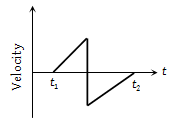(2)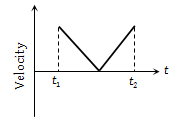(3)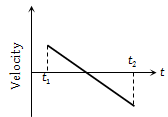(4)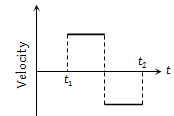Concept Questions :-

Work-Energy theorem
High Yielding Test Series + Question Bank - NEET 2020

Difficulty Level:

The relationship between force and position is shown in the figure given (in one dimensional case). The work done by the force in displacing a body from x = 1 cm to x = 5 cm is -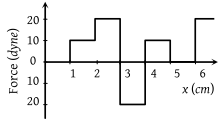(1) 20 ergs

(2) 60 ergs

(3) 70 ergs

(4) 700 ergs

Concept Questions :-

Work done by variable force
High Yielding Test Series + Question Bank - NEET 2020

Difficulty Level:

The pointer reading v/s load graph for a spring balance is as given in the figure. The spring constant is-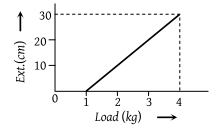(1) 0.1 N/cm

(2) 5 N/cm

(3) 0.3 N/cm

(4) 1 N/cm

Concept Questions :-

Elastic potential energy